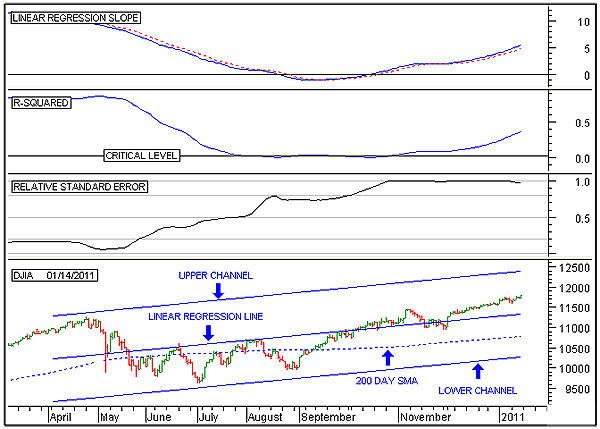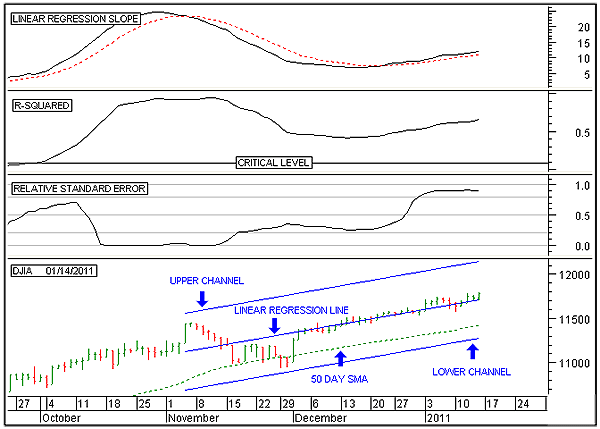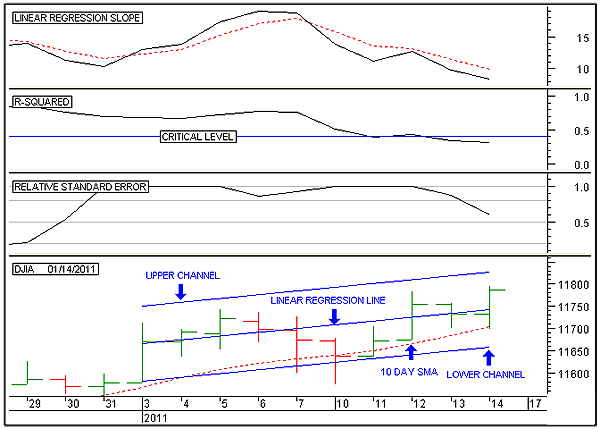HOT TOPICS LIST

INDICATORS LIST

LIST OF TOPICS

# The DJI ETF Nears Long-Term Reversal

01/17/11 01:30:22 PM
by Alan R. Northam

Statistical analysis is used here to show that the uptrend of the Dow Jones Industrial Average is in its final stage.

Security:   .DJI
Position:   N/A

 Figure 1 shows the long-term analysis of the Dow Jones Industrial Average exchange traded fund (ETF) (DIA). On the price chart is shown the 200-day linear regression line along with its two standard deviation upper and lower channel lines. Statistically, these two channel lines contain 95% of all trading activity. Thus, the upper channel line represents a strong line of resistance and the lower channel line as a strong line of support. The middle line is the linear regression trendline and represents the median price. A move in price above the linear regression line represents overzealous buyers, while a move below represents overzealous sellers. When the price moves too far away from the linear regression trendline, it generally works its way back. Thus, the linear regression line acts as a price magnet, attracting price to it. Note that the linear regression line is sloping in an upward direction, indicating that the long-term trend is upward.FIGURE 1: DJIA, DAILY. This chart shows the daily price chart of the Dow Jones Industrial Average in the bottom panel along with the 200-day linear regression line and the upper and lower linear regression channel lines, the linear regression slope indicator in the top panel, the R-squared indicator in the second panel, and the relative standard error indicator in the third. Graphic provided by: MetaStock. The relative standard error indicator is a measure of how close to or how far away price has wandered from the linear regression Line and is a measure of volatility. When price is equal to the same value as the linear regression line the standard error is zero When price moves away from the linear regression line the standard error increases. The relative standard error indicator moves between zero and one with zero representing no volatility in price and one representing high volatility. High volatility normally occurs near or at the end of a trend. Once volatility becomes high we can expect that the end of the trend is near. Note the current high volatility in price. This is an indication that the long term upward rally is in its final stage. The linear regression slope indicator is a measure of price momentum. This indicator is currently above its zero line and pointing upward, indicating that the price is accelerating upward. Price acceleration is an indication of a strong uptrend. Price is usually either accelerating or decelerating. When price is accelerating, it is an indication of a strong trend, and when it is decelerating, it is a sign that the trend is nearing its completion.The R-squared indicator is a measure of the strength of the trend. When this indicator is above its critical level, it indicates that a strong trend is in place. What is different in this indicator from other indicators of price strength is that when it is above its critical level, it indicates a 95% probability that the current trend will continue. The critical level is different for differing time periods. For long time periods, the critical level is low and moves upward as the time period shortens. The calculation of this critical level involves a complex statistical calculation. Generally, when the R-squared indicator falls below its critical level, a change in trend soon occurs.In Figure 1, I have shown the daily price bars and three indicators. The normal progression of these three indicators in determining a change in trend is that volatility becomes very high, as indicated by the relative standard error indicator. Once volatility reaches a high state, the trend starts to weaken as indicated by the R-squared indicator falling below its critical level. Following the weakening of the existing trend, the trend itself finally reverses, as indicated by the linear regression slope indicator crossing to the other side of its zero line. In analyzing the long-term trend of the DJIA, it is noted that volatility is extremely high, indicating that the end of the long-term trend is nearing. However, the current uptrend remains strong for now, since the R-squared indicator remains above its critical level. Further, price continues to accelerate, as noted by the linear regression slope indicator pointing upward. While volatility remains extremely high, the breakdown in price has not yet started. However, with volatility being extremely high, we can be confident that a reversal in the long-term trend could occur in the months ahead. Figure 2 shows the intermediate-term analysis of the DJIA. On the price chart is shown the 50-day linear regression line along with its two standard deviation upper and lower channel lines, representing intermediate levels of support and resistance. Note that the linear regression trendline is sloping upward, indicating that the intermediate-term trend is upward. The relative standard error ndicator indicates high volatility.The R-squared indicator is currently above its critical level, indicating that a strong uptrend is in force with a 95% probability that the current trend will continue in the future.The linear regression slope indicator indicates that price continues to accelerate, signaling that the uptrend remains healthy and strong.The intermediate-term analysis shows volatility has risen to a high level, completing the first step to a trend reversal. However, the intermediate-term trend remains strong and price continues to accelerate, indicating that the trend has not yet started to break down. However, with high volatility we should expect a reversal in the intermediate-term trend to occur in the weeks ahead.FIGURE 2: DJIA, DAILY. This chart shows the daily price chart of the Dow Jones Industrial Average in the bottom panel along with the 50-day linear regression line and the upper and lower linear regression channel lines, the linear regression slope indicator in the top panel, the R-squared indicator in the second panel, and the relative standard error indicator in the third. Graphic provided by: MetaStock. Figure 3 shows the short-term analysis of the DJIA. On the price chart is shown the 10-day linear regression line along with its two standard deviation upper and lower channel lines, representing short-term levels of support and resistance. Note that the linear regression trendline is sloping upward, indicating that the short-term trend is upward. The relative standard error indicator indicates above-average volatility, thus completing the first step to a short-term trend reversal. Note that when the standard error indicator is above 0.5 but below 0.8, above-average volatility is indicated with high volatility indicated above 0.8. Further, when below 0.5 and above 0.2, below-average volatility is indicated with low volatility indicated below 0.2.The R-squared indicator is currently just slightly below its critical level, indicating a weak uptrend and completing the second step to a short-term trend reversal.The linear regression slope indicator is currently moving downward, signaling price deceleration. Price deceleration normally occurs just before a reversal in trend.The short-term analysis shows that a reversal in the short-term trend is just days away. Short-term trend reversals are normally short-term corrections to the ongoing longer-term uptrend. However, all long-term reversals in trend also start with a short-term reversal.FIGURE 3: DJIA, DAILY. This chart shows the daily price chart of the Dow Jones Industrial Average in the bottom panel along with the 10-day linear regression line and the upper and lower linear regression channel lines, the linear regression slope indicator in the top panel, the R-squared indicator in the second panel, and the relative standard error indicator in the third. Graphic provided by: MetaStock. In conclusion, the DJIA currently remains in a long-term uptrend. However, this long-term trend is most likely in its final stage and we should expect to see a reversal in the trend in the months ahead. The intermediate-term trend is also in its final stage and we should expect a reversal in the weeks ahead. Over the short term, we should expect at least a price correction in the days ahead. However, with volatility being high in both the long term and the intermediate term, we may experience that this potential short-term correction could turn into a possible long-term decline.

Alan R. Northam

Alan Northam lives in the Dallas, Texas area and as an electronic engineer gave him an analytical mind from which he has developed a thorough knowledge of stock market technical analysis. His abilities to analyze the future direction of the stock market has allowed him to successfully trade of his own portfolio over the last 30 years. Mr. Northam is now retired and trading the stock market full time. You can reach him at inquiry@tradersclassroom.com or by visiting his website at http://www.tradersclassroom.com. You can also follow him on Twitter @TradersClassrm.

 Comments or Questions? Article Usefulness 5 (most useful) 4 3 2 1 (least useful)